## 教与学优化（TLBO）算法

Rao 等人 (2011) 开发了 TLBO 算法，该算法不需要调整任何算法相关（algorithm-specific）参数。该算法描述了两种基本的学习模式：(i) 通过教师学习（称为教师阶段）；(ii) 通过与其他学员的交流学习（称为学员阶段）。在该优化算法中，一组学员被认为是群体，而提供给学员的不同学科被认为是优化问题的不同设计变量，学员的成绩与优化问题的“适应度”值类似。在整个群体中最好的解被认为是老师。设计变量实际上是给定优化问题的目标函数中涉及的参数，最佳解是目标函数的最佳值。

TLBO 的工作分为“老师阶段”和“学员阶段”两部分。下面将解释这两个阶段的工作 (Rao 2016a)。

### 教师阶段

T_F并非 TLBO 算法的参数，其值不作为算法的输入，而是由算法使用方程 (2) 随机决定。在对许多基准函数进行了大量实验之后，得出的结论是，如果 $T_F$ 的值在 1 和 2 之间，则算法性能好。而且，如果 $T_F$ 值为 1 或 2，则发现算法性能更好，并且因此为了简化算法，建议教学因子取 1 或 2。基于 $\text{Difference_Mean}_{j, k, i}$，在教师阶段根据下式更新现有解。

### 学员阶段

TLBO 算法的流程图如下所示。TLBO 算法的最大函数评估次数 = 2 $\times$ 种群规模 $\times$ 迭代次数。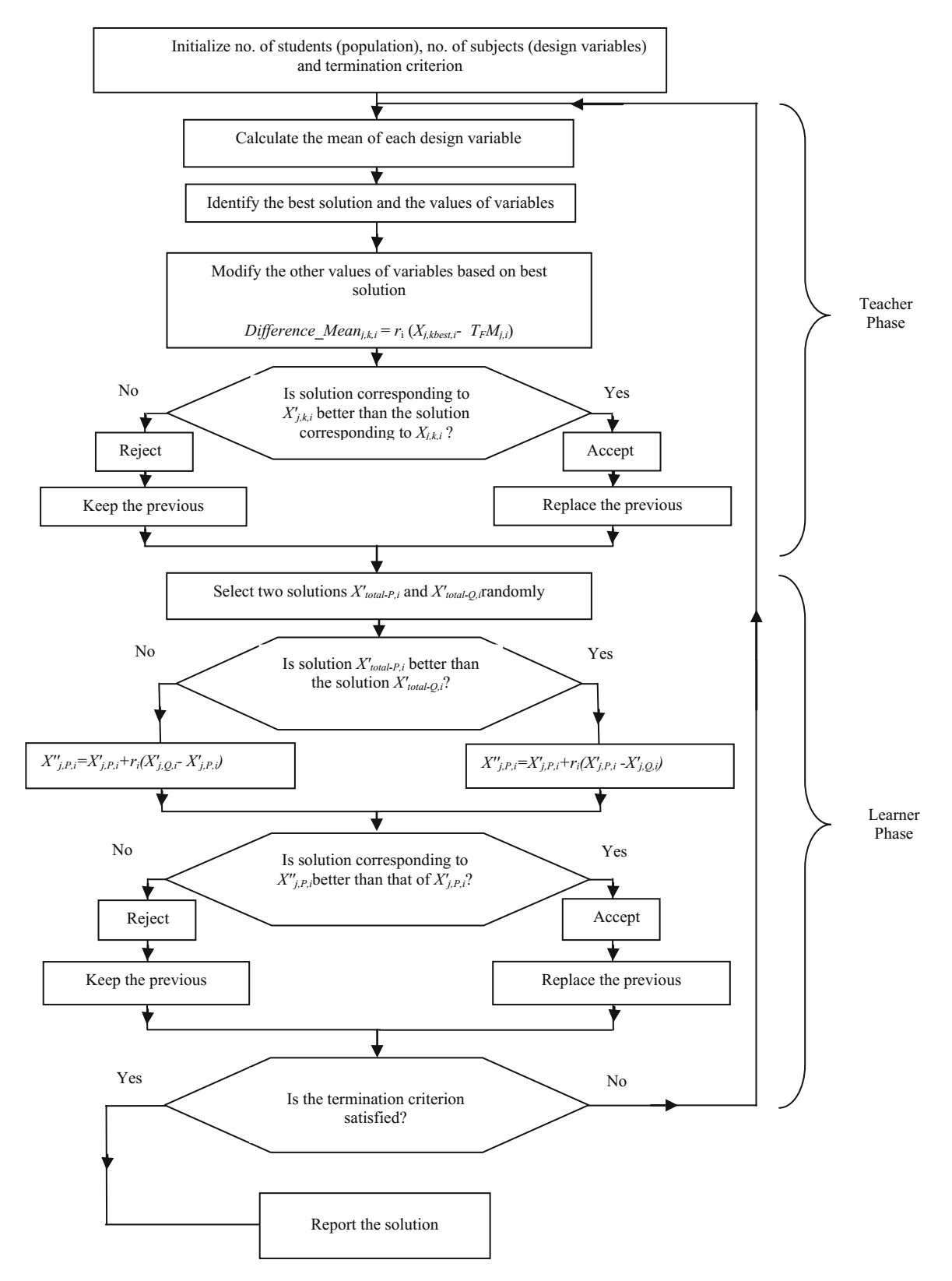## 非支配排序教与学优化（NSTLBO）算法

NSTLBO 算法是为解决多目标优化问题而开发的。NSTLBO 算法是 TLBO 算法的扩展。这是一种解决多目标优化问题的后验（posteriori）方法，能够保留了多个不同的解集。NSTLBO 算法类似于 TLBO 算法，由教师阶段和学员阶段组成。为了处理多个目标，NSTLBO 算法有效地与非支配排序（non-dominated sorting）方法和拥挤距离（crowding distance）计算相结合。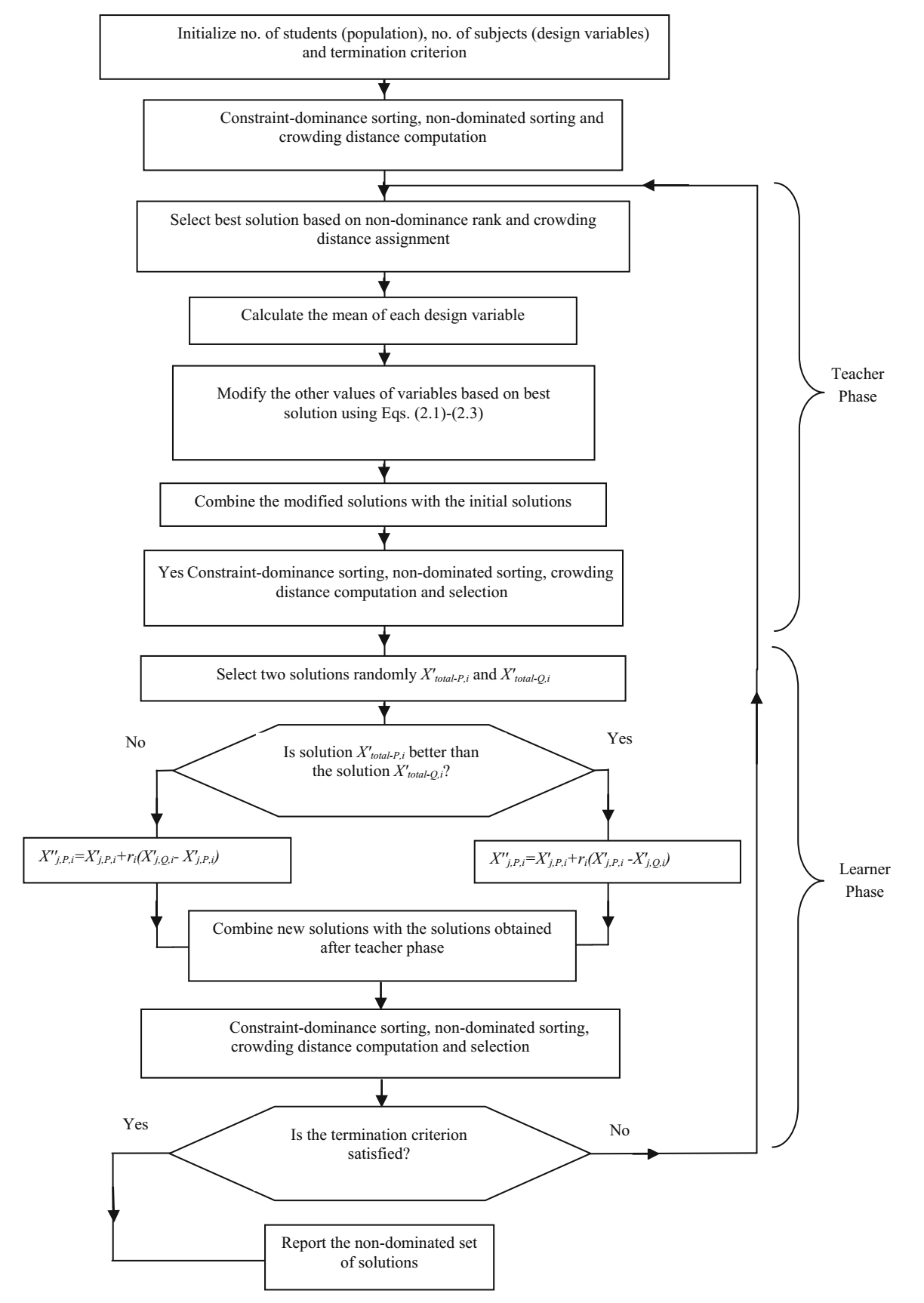### 拥挤距离的计算

1. 确定前沿 $F$ 中解的数量 $l = \vert F \vert$。为集合中每个解 $i$ 分配 $CD_i = 0$
2. 对每个目标函数 $m = 1, 2, \cdots, M$，按 $f_m$ 的最差序对集合进行排序。
3. 对于 $m = 1, 2, \cdots, M$，将最大拥挤距离分配给排序列表中的边界解（$CD_1 = CD_l = \infty$），对于排序列表 $j = 2$$(l - 1)$ 的所有其他解，拥挤距离如下：

## Jaya 算法

Rao (2016b) 开发了 Jaya 算法，该算法易于实现，不需要调整任何算法相关的参数。在 Jaya 算法中，$P$ 个初始解按照过程变量的上下界随机生成。此后，使用等式 (9) 随机更新每个解。$f$ 是要最小化（或最大化）的目标函数。有 $d$ 个设计变量。最好解对应的目标函数值为 $f\_best$，最坏解对应的目标函数值为 $f\_best$

Jaya 算法的流程图如下图所示 (Rao et al. 2017)。对于每个候选解，Jaya 算法仅在每代中评估目标函数一次。因此，Jaya 算法所需的函数评估总次数 = 种群规模 $\times$ 迭代次数。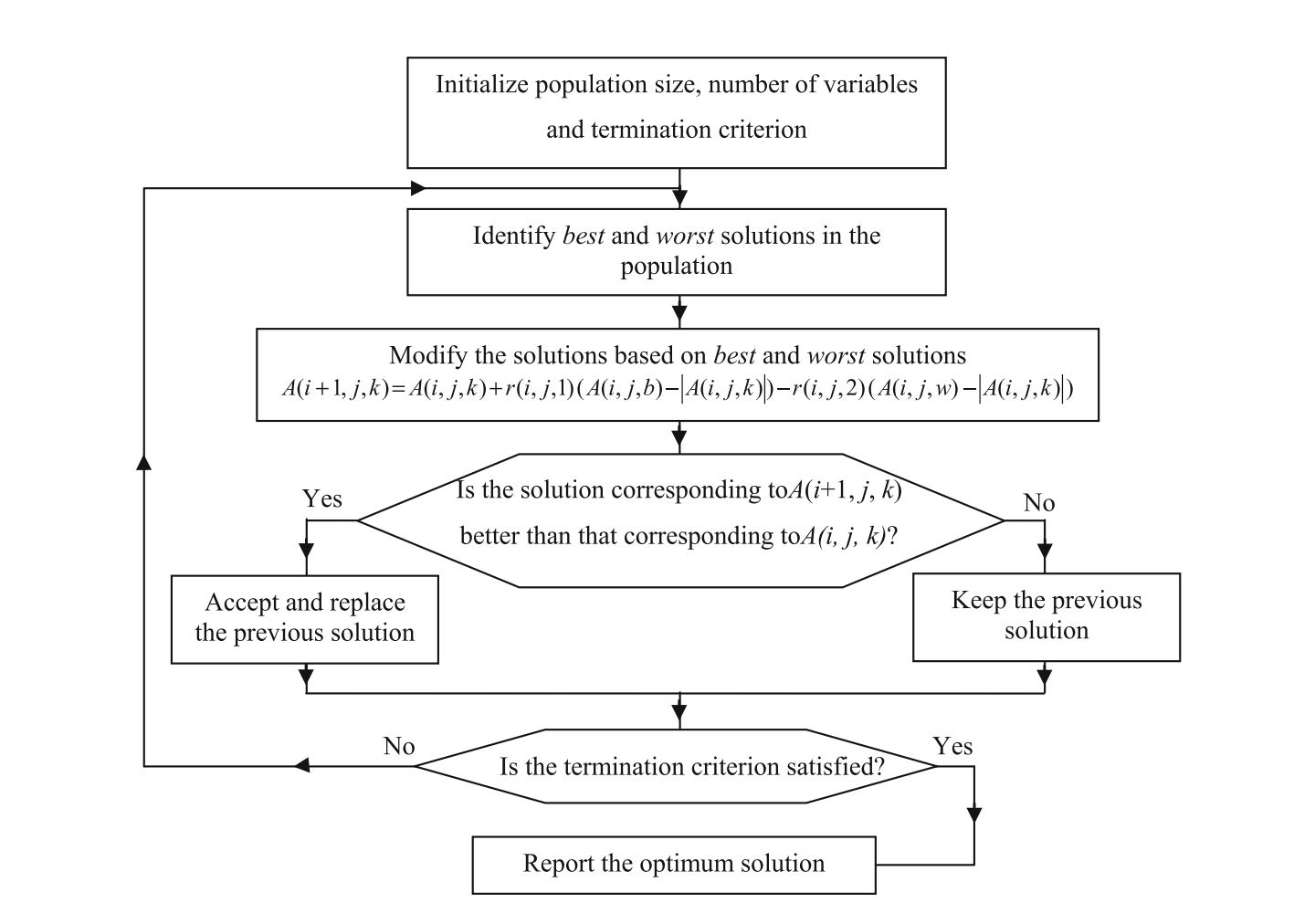### 无约束优化问题的 Jaya 算法演示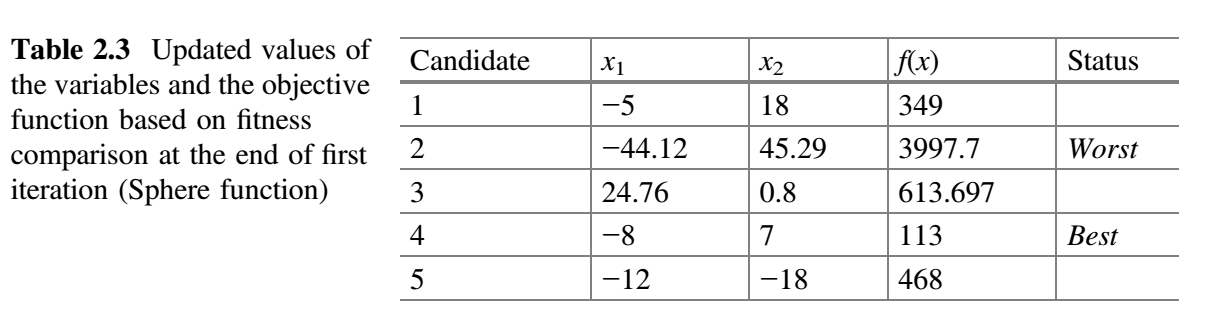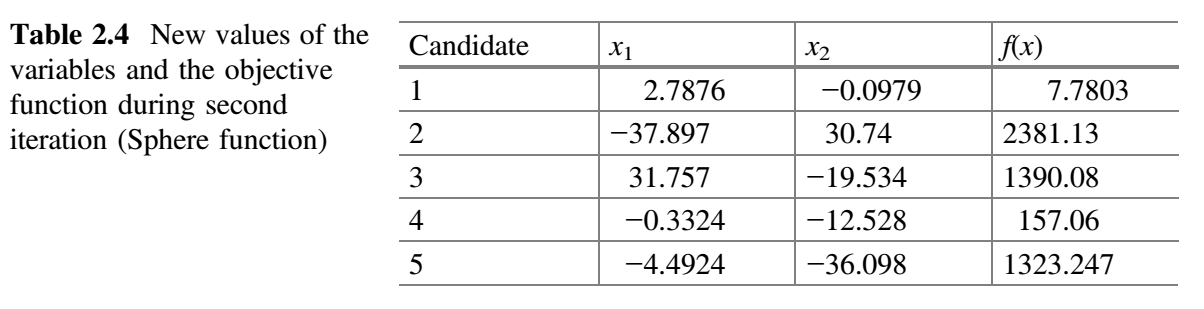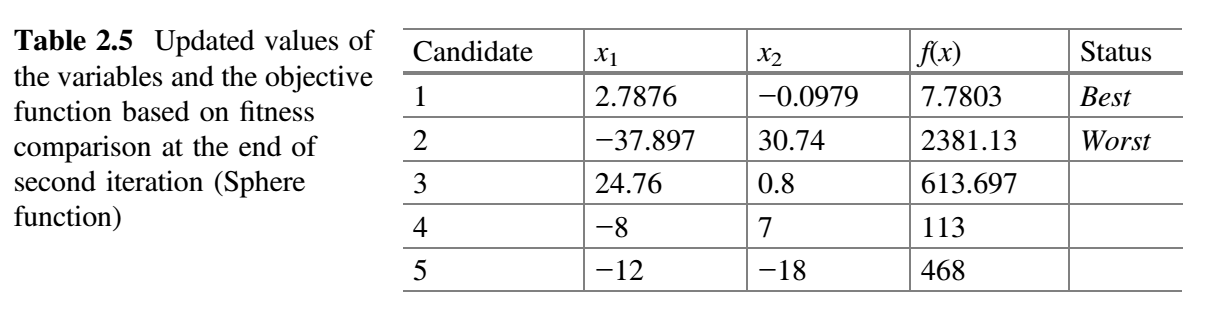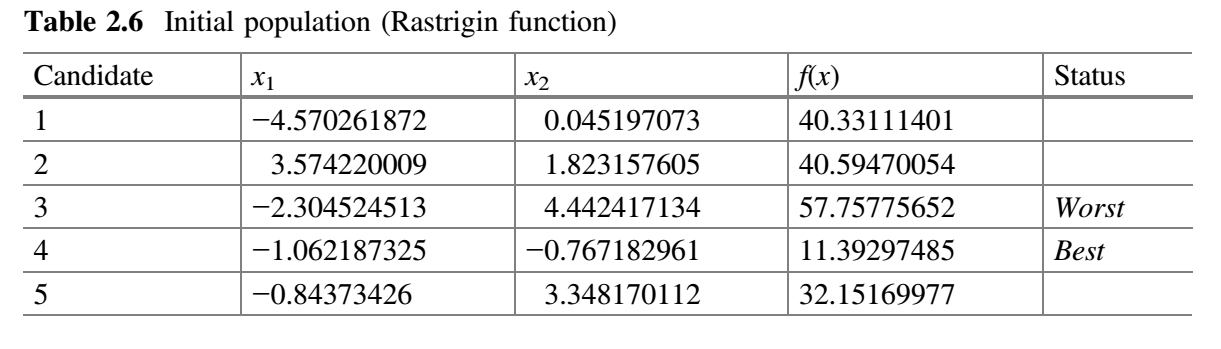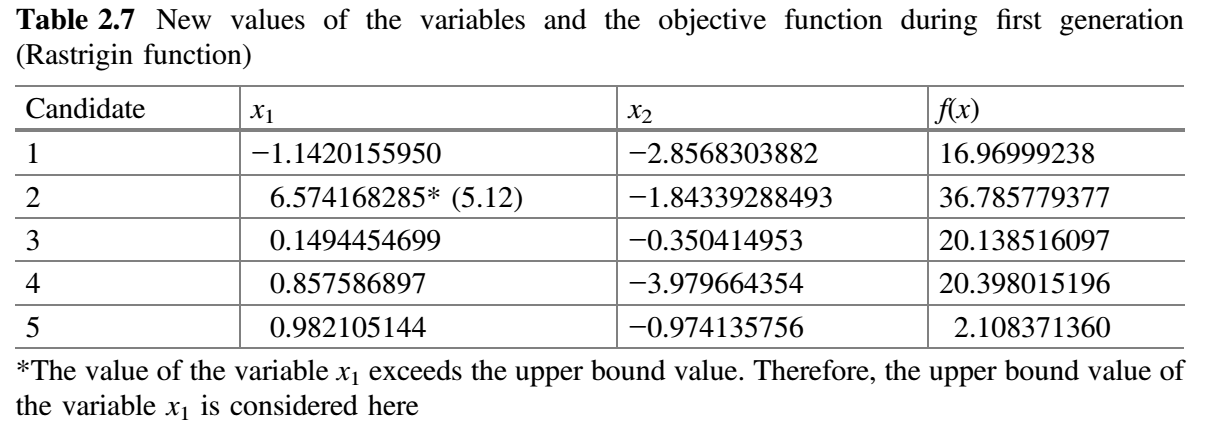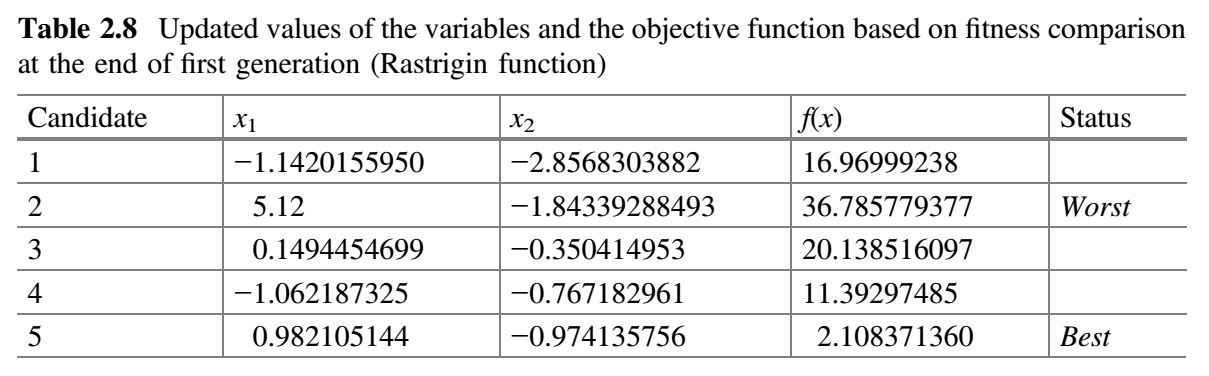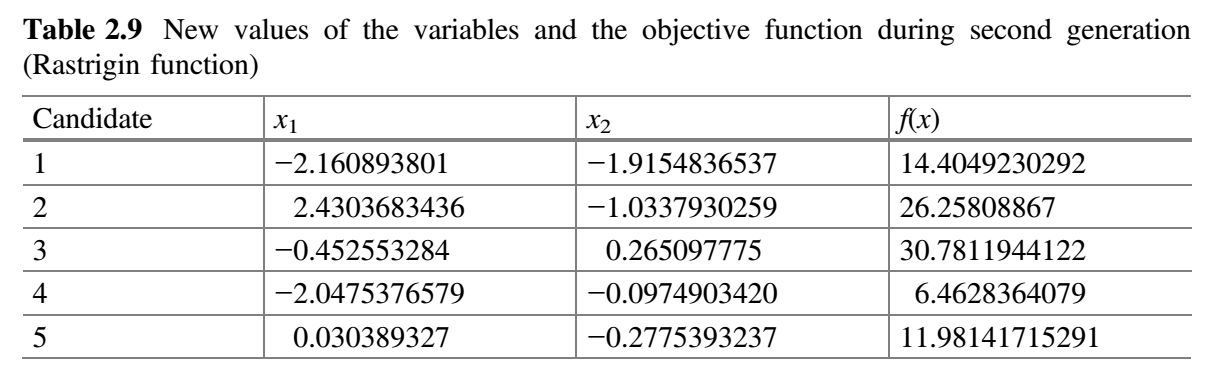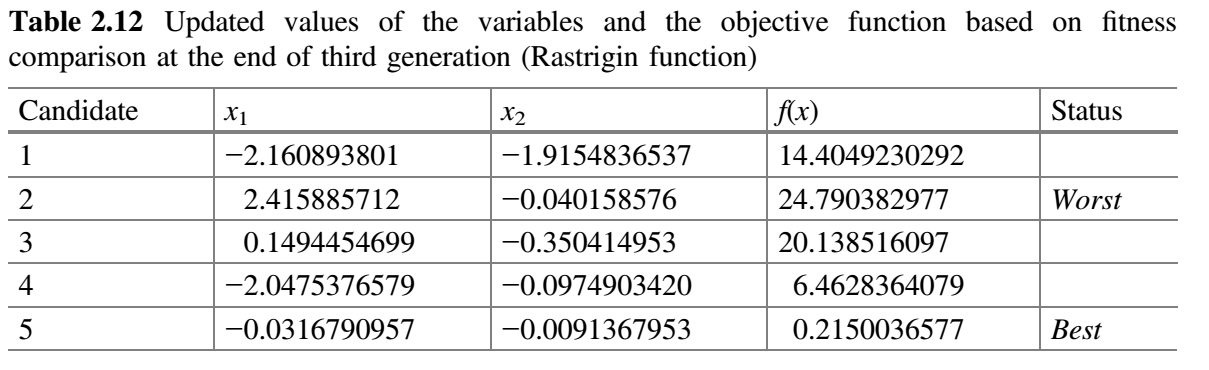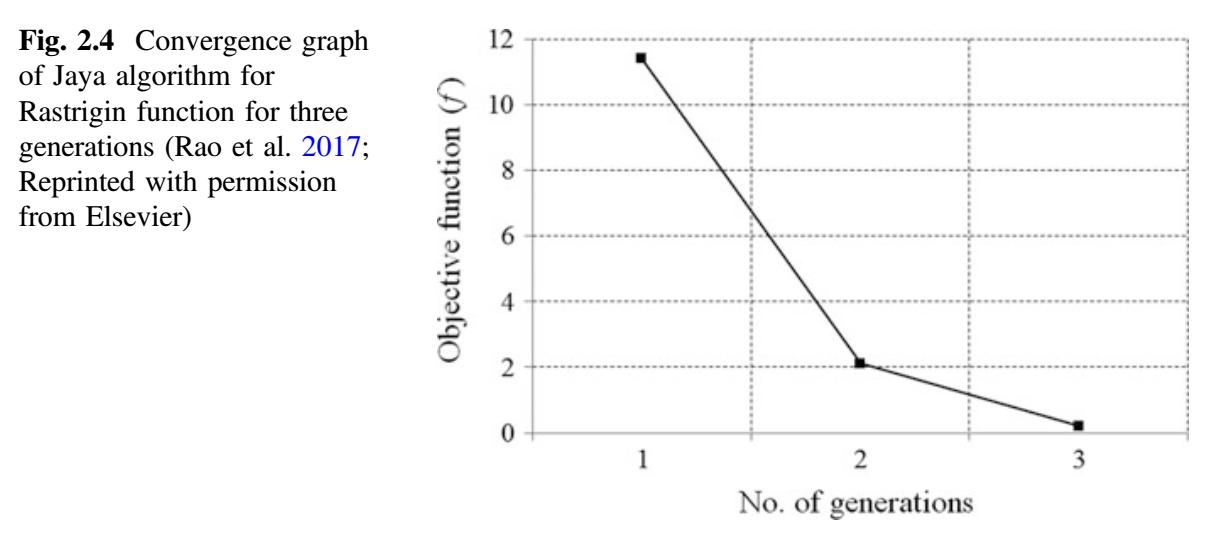### 约束优化问题的 Jaya 算法演示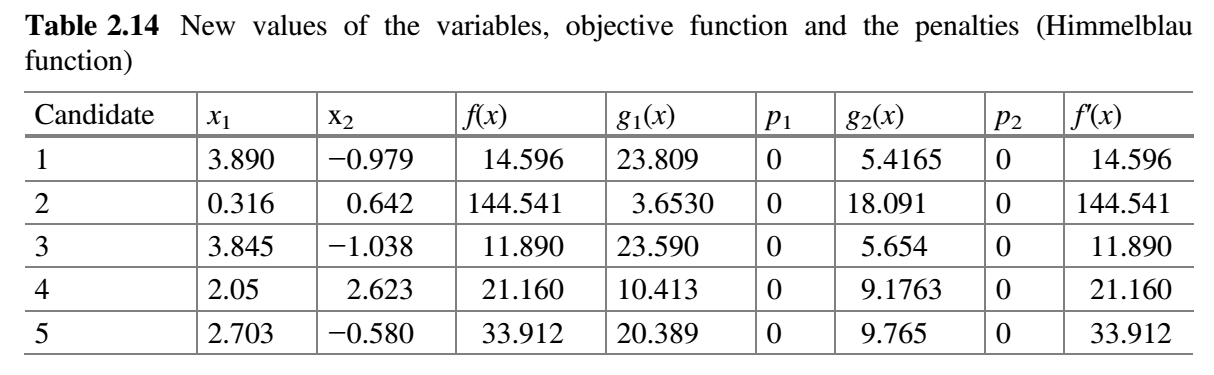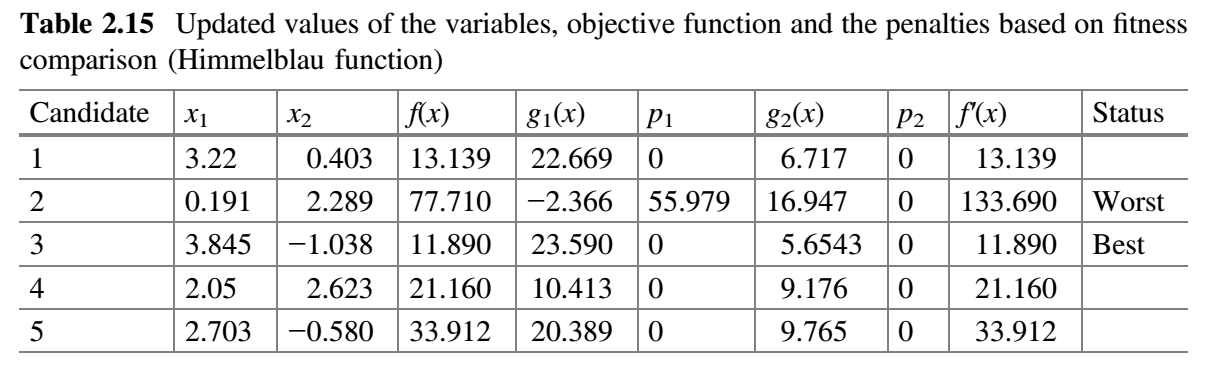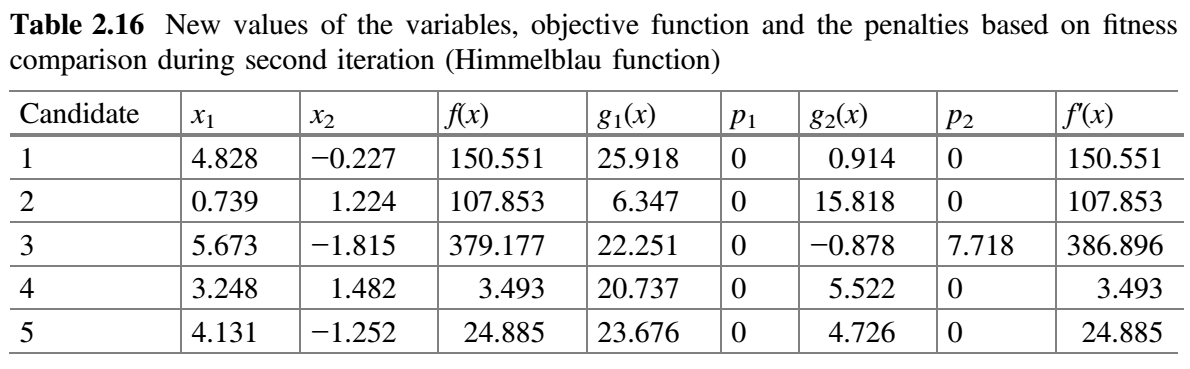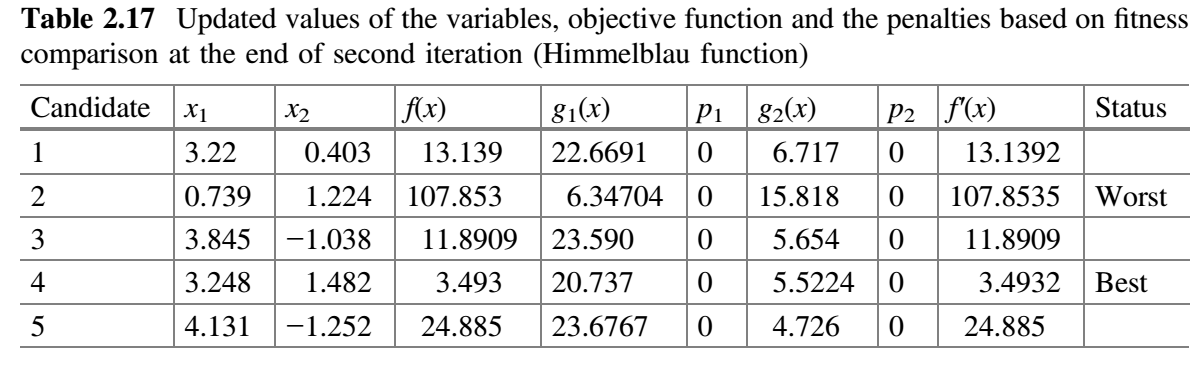## 自适应 Jaya 算法## 基于拟反向的 Jaya（QO-Jaya）算法

$i = 1, 2, 3, \dots, g$，$j = 1,2,3,\dots,P$$k = 1,2, 3, \dots, d$。其中，$A_k^L$$A_k^U$ 是第 $k$ 个变量的下限值和上限值，$A_{i,j,k}^q$$A_{i,j,k}$ 的拟反向值。例如，如果变量的下限和上限分别为 10 和 60，且当前迭代中变量 $A_{i,j,k}$ 的值为 23，则 $a = 35$（给定范围的中值）， $b = 10 + 60 - 23 = 47$。事实上，完全反向值（镜像点）是 47。而变量的拟反向值 $A_{i,j,k}^q = rand(35, 47)$。这意味着，将会生成 35 到 47 之间的任何值。下图给出了 QO-Jaya 算法的流程图 (Rao and Rai 2017a, b)。 QO-Jaya 算法所需的函数评估次数 = 种群规模 $\times$ 迭代次数。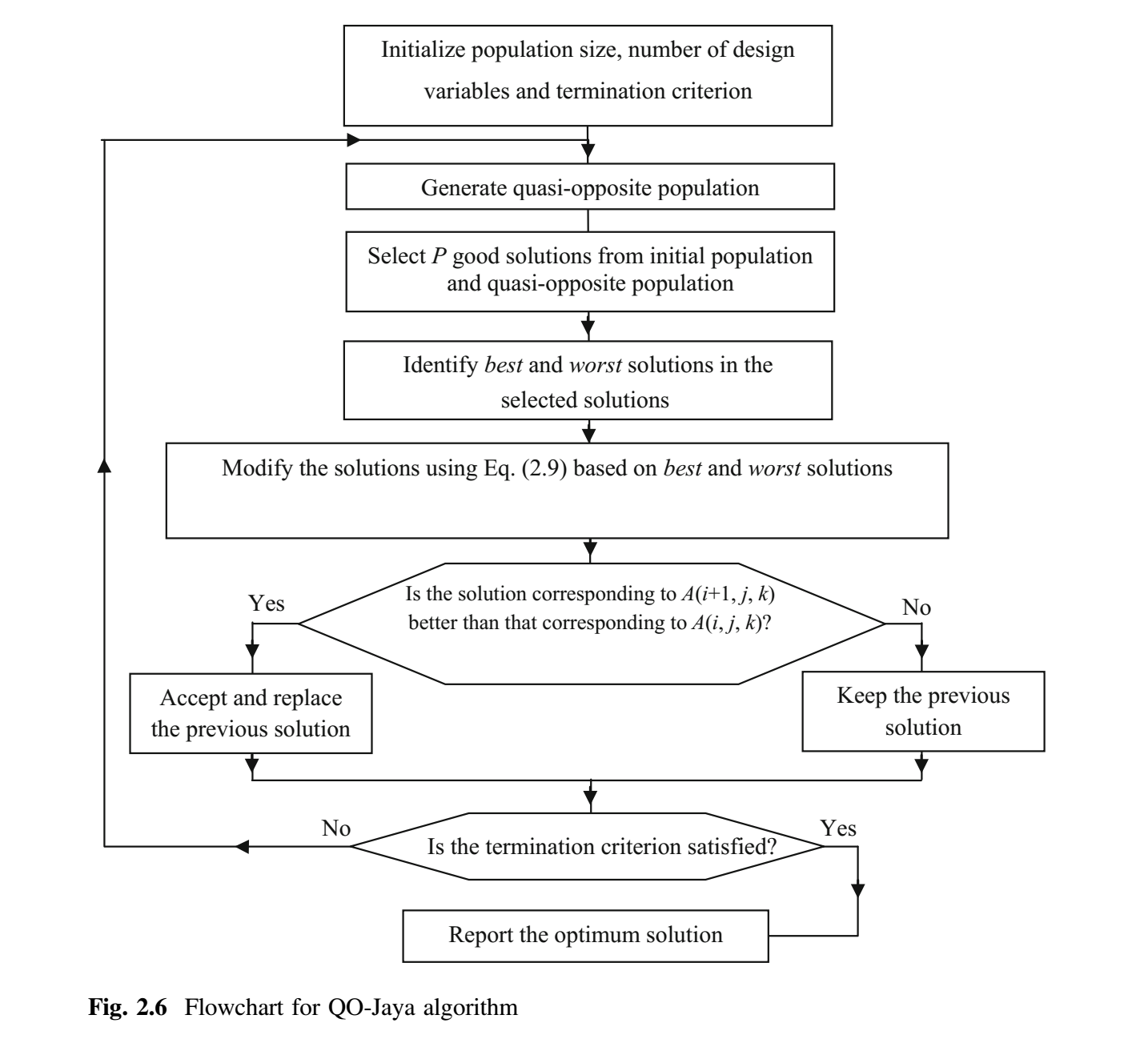## 自适应多种群（SAMP）Jaya 算法

• 提出的 SAMP-Jaya 算法基于解的质量将整个群体划分为多个子群。将子群分布到整个搜索空间而不是集中于某个区域。因此提出的算法被期望产生最优解。
• 搜索过程中，SAMP-Jaya 算法基于问题景观的变化强度来修改子群的数量。这意味着子群的数量会增加或减少。在 SAMP-Jaya 算法中, 子群的数量基于解的强度变化自适应地进行修该。此特性支持搜索过程中追踪最优解，提高了搜索过程的多样性。此外，重复解被新产生的解替代以维持多样性和增强探索过程。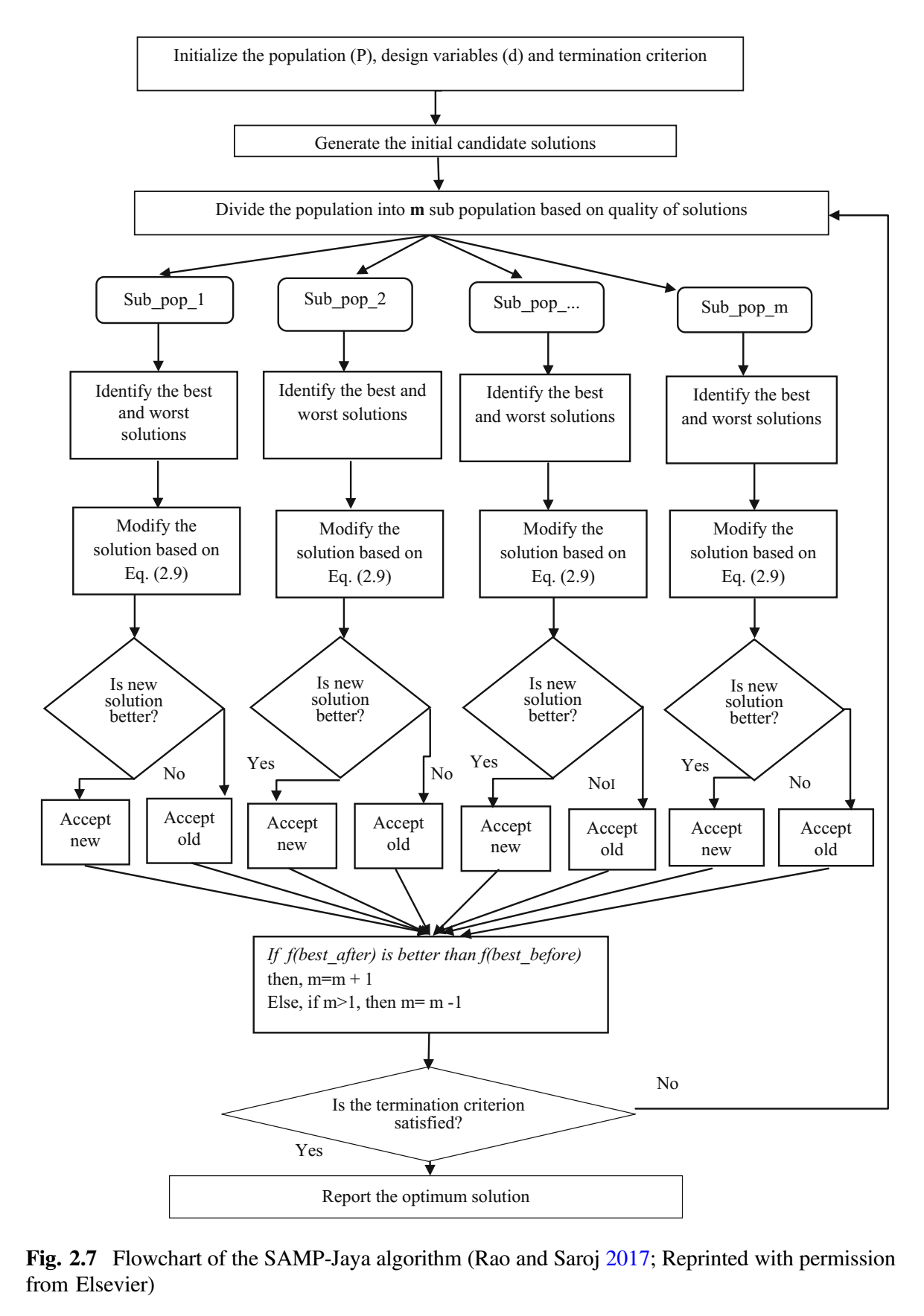and Saroj 2017)。SAMP-Jaya 算法的基本步骤如下：

1. 从设置设计变量 $d$、种群数量 $P$ 和停止准则开始。
2. 下一步是基于定义的适应度函数（对于特定的问题），计算初始解。
3. 根据解的质量, 将整个种群划分为 $m$ 个子群（最初考虑 $m=2$）。
4. 每个子群都独立地使用 Jaya 算法修改（更新）子群中的解。接受修改的解，当且仅当他比旧解好。
5. 合并所有子群。检查 $f(best \_ before)$ 是否优于 $f(best \_ after)$。这里，$f(best \_ before)$ 是之前整个子群最佳解，$f(best \_ after)$ 是当前整个种群的最佳解。如果 $f(best \_ after)$ 的值优于 $f(best \_ before)$ 的值，则 $m$ 加 1（即 $m = m + 1$），目的是增加搜索过程的探索特征。否则，$m$ 减 1（即 $m = m - 1$），因为算法需要更多的开发能力。
6. 检查停止条件。如果已达到终止条件，则结束循环并给出最佳解。否则，(a) 用随机生成的解替换重复的解；(b) 按步骤 3 重新划分子群并重新执行后续步骤。

### 无约束优化问题的 SAMP-Jaya 算法演示SAMP-Jaya 算法的各个步骤给出如下：

1. 设计变量数 $d = 2$、种群规模 $P = 20$、子群（初始）= 2、终止准则 = 2 次迭代。
2. 下一步是计算问题的初始解。
3. 根据解的质量，将整个种群分为 $m$ 组（初始考虑 $m = 2$）。
4. 每个子群独立地使用 Jaya 算法更新子群中的解。每个修改解只有在优于旧解时才被接受。表 2.20a、2.20b 分别展示了用于子群 1 和 2 的随机数。现在，比较表 2.19a 和 2.21a，更新值（即更好的值）保留在表 2.22a 中。类似地，比较表 2.19b 和 2.21b，更新值（即更好的值）保留在表 2.22b 中。
5. 合并所有子群。表 2.23 显示了第一次迭代结束时合并的种群。
检查 $f(best \_ before)$ 是否比 $f(best \_ after)$ 好。这里，$f(best \_ before)$ 是先前整个种群的最佳解，$f(best \_ after)$ 是当前整个种群的最佳解。在第一次迭代结束时，发现的 $f(best \_ after)$ 值比 $f(best \_ before)$ 的值好，因此 $m$ 加 1（即 $m = 2 + 1 = 3$），其目的是增加搜索过程的探索特征。
6. 检查终止条件。如果达到终止条件，则终止循环并报告最佳解。由于在本示例中终止条件被定为 2 次迭代，因此该过程跳到步骤 3 而并重复（表 2.24）。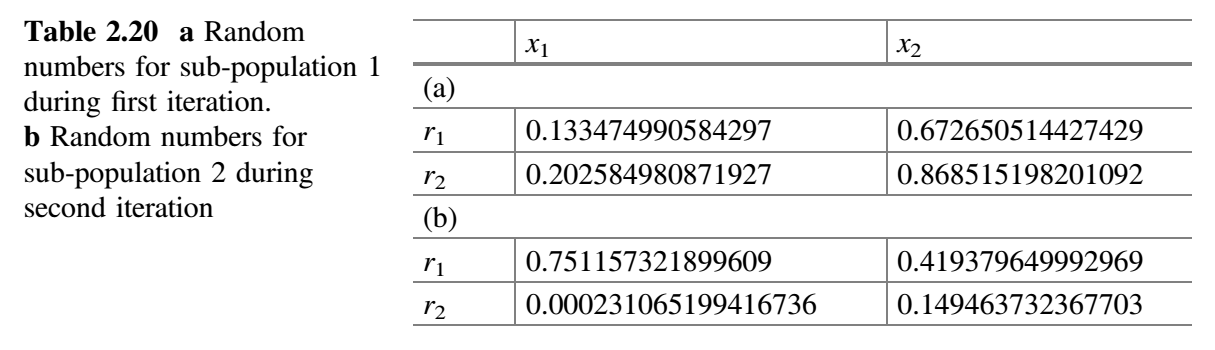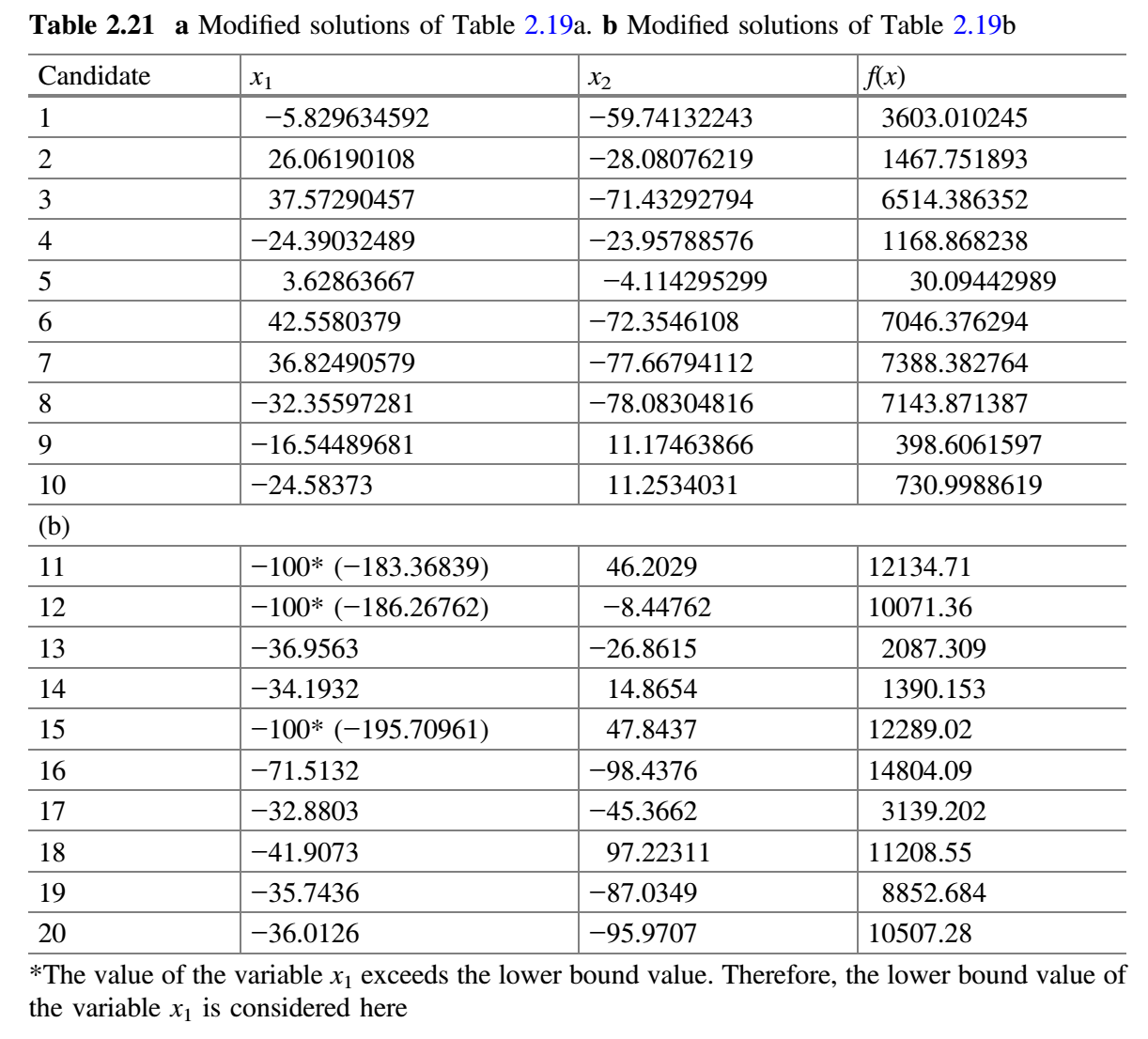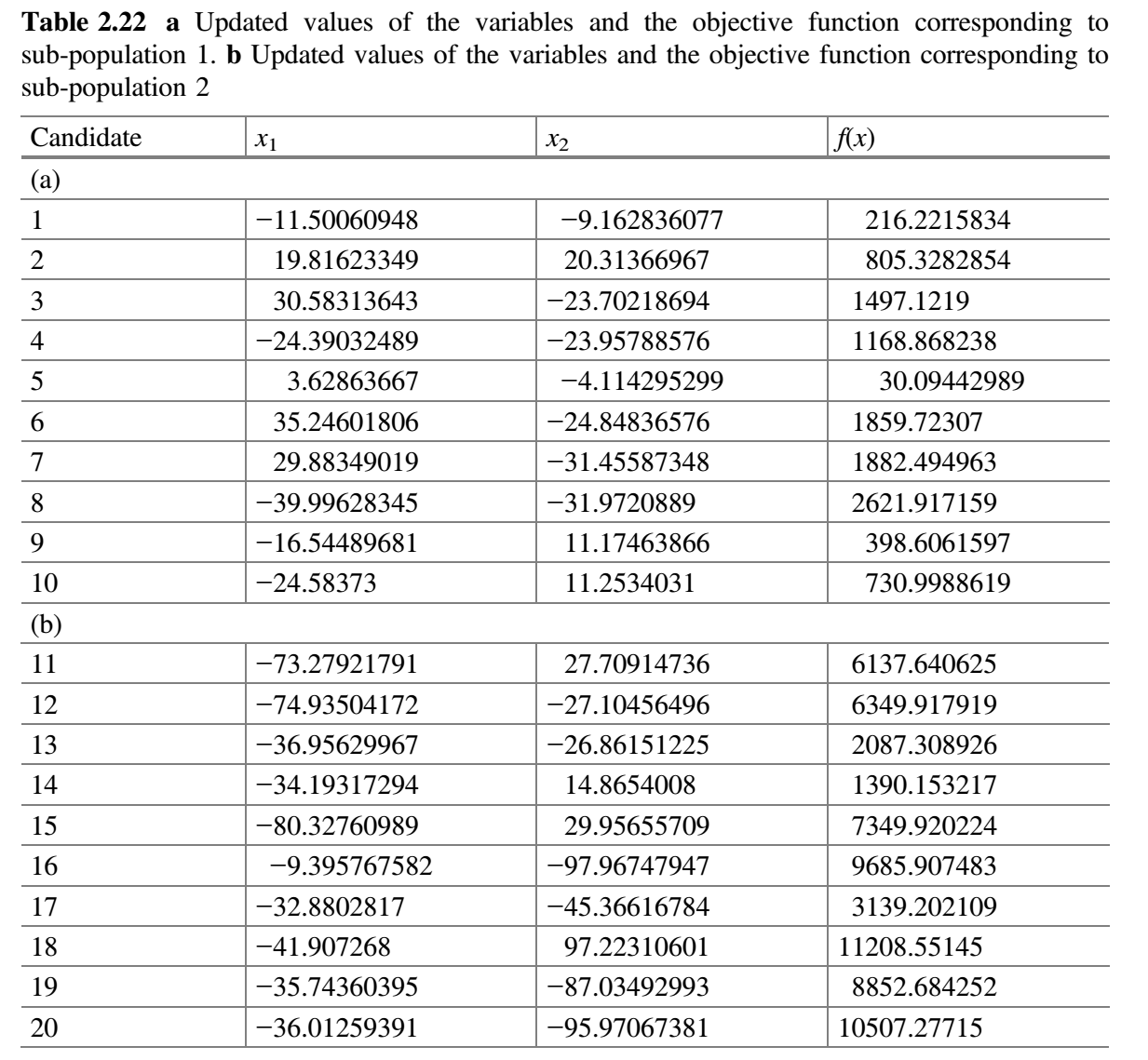1. 根据解的质量，将整个种群分成 3 组。
2. 每个子群独立使用 Jaya 算法更新解。修改的解只有在优于旧解时才被接受。表 2.25a-c 分别显示了用于子群 1、2 和 3 的随机数。比较表 2.24a 和 2.26a，更新值保存在表 2.27a 中。同样，比较表 2.24b 和 2.26b，更新值保存在表 2.27b 中。同样，比较表 2.24c 和 2.26c，更新值保存在表 2.27c 中。
3. 合并所有子群。表 2.28 显示了第二次迭代结束时合并的种群。检查 $f(best \_ after)$ 是否比 $f(best \_ before)$ 好。在第二次迭代结束时，发现 $f(best \_ after)$ 的值比 $f(best \_ before)$ 的值好，因此 $m$ 加 1（即 $m = 3 + 1 = 4$）。
4. 检查停止条件。如果达到停止条件，则终止循环并报告最佳解。否则，该过程将继续，直到达到终止标准。在本示例中，终止条件被定为 2 次迭代，因此该过程被终止。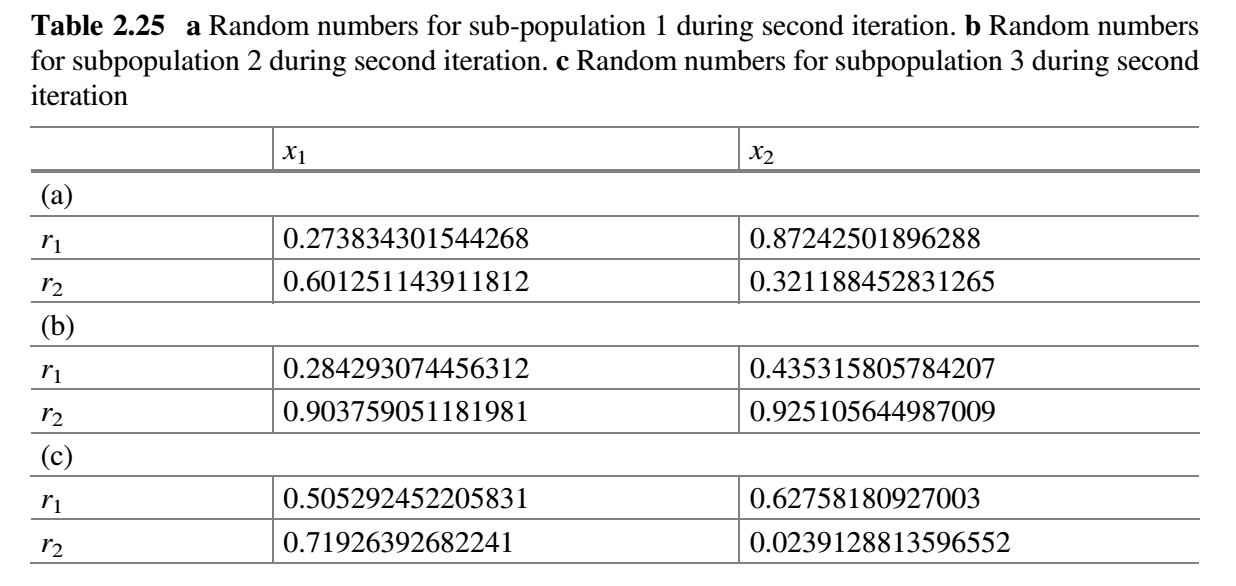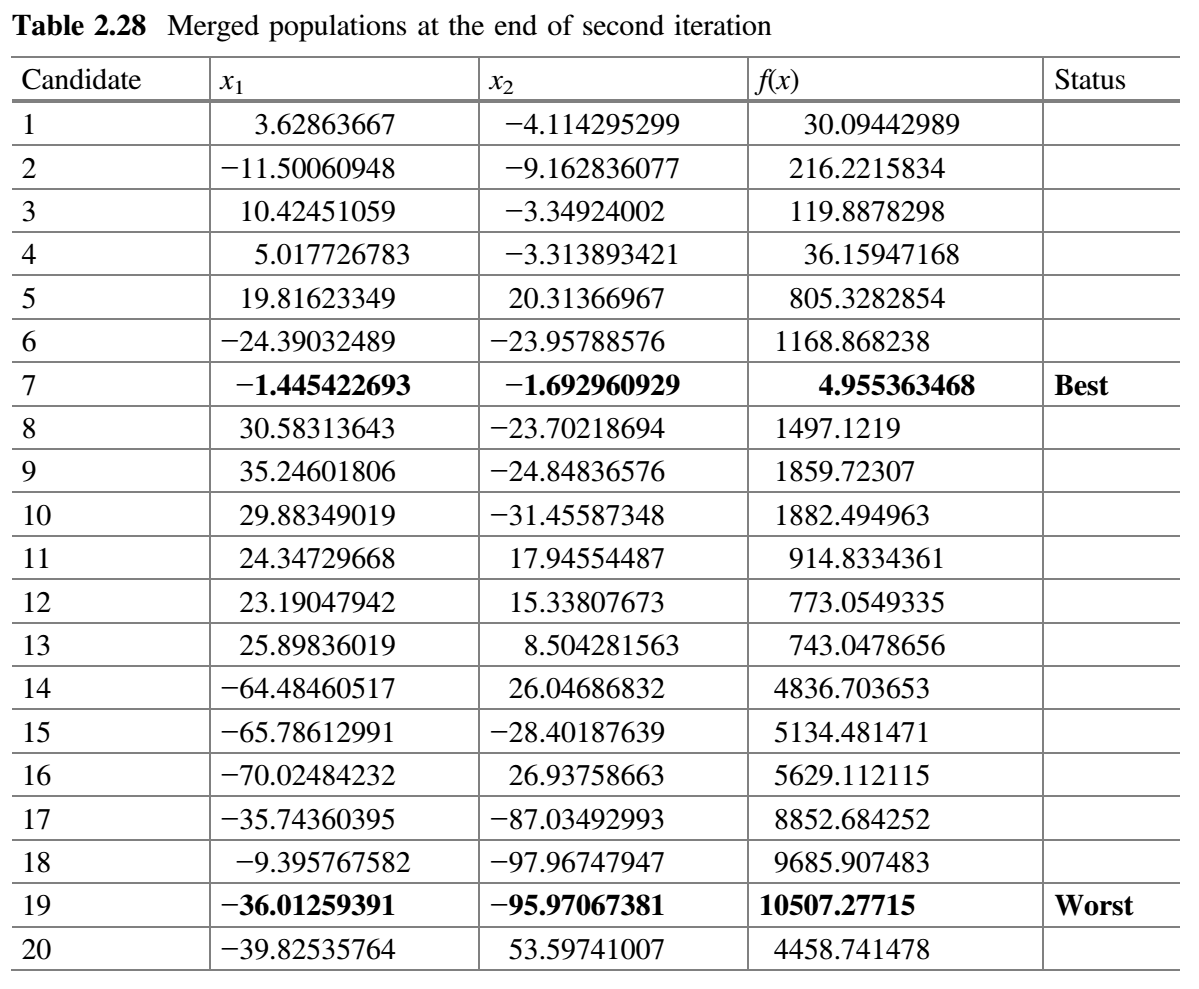## 自适应多种群精英策略（SAMPE）Jaya 算法

SAMPE-Jaya 算法对基本 Jaya 算法进行了以下修改：

• 提出的算法基于解的质量将种群划分为多个子群。此外，劣势群体（适应度值低的群体）的最差解被优势群体（适应度值高的群体）的解（精英解）所取代。使用多个子群将解分布于搜索空间，而不是集中在特定区域。因此，该算法可望产生最优解，并监测问题景观的变化。
• 在搜索过程中，SAMPE-Jaya 算法根据监测到的问题景观的变化强度修改子群的数量。此外，为了保持多样性和加强探索过程，用新生成的解替换重复的解。

SAMPE-Jaya 算法的基本步骤如下：

1. 从设计变量数量 $d$、种群规模 $P$、精英规模 $ES$ 和终止标准的设置开始。
2. 下一步是根据问题定义函数计算初始解。
3. 根据解的质量，将整个种群分为 $m$ 组，用精英解代替劣势解（与 $ES$ 相等）。
4. 每个子群体独立使用 Jaya 算法修改解。修改的解只有在优于旧解时才会保留。
5. 合并使用子群。检查 $f(best \_ before)$ 是否优于 $f(best \_ after)$。这里，$f(best \_ before)$ 是之前整个子群最佳解，$f(best \_ after)$ 是当前整个种群的最佳解。如果 $f(best \_ after)$ 的值优于 $f(best \_ before)$ 的值，则 $m$ 加 1（即 $m = m + 1$），目的是增加搜索过程的探索特征。否则，$m$ 减 1（即 $m = m - 1$），因为算法需要更多的开发能力。
6. 检查终止条件。如果搜索过程达到停止标准，则终止循环并报告最佳解。否则，(a) 用随机生成的解替换重复解；(b) 按照步骤 3 重新划分子群，然后执行后续步骤。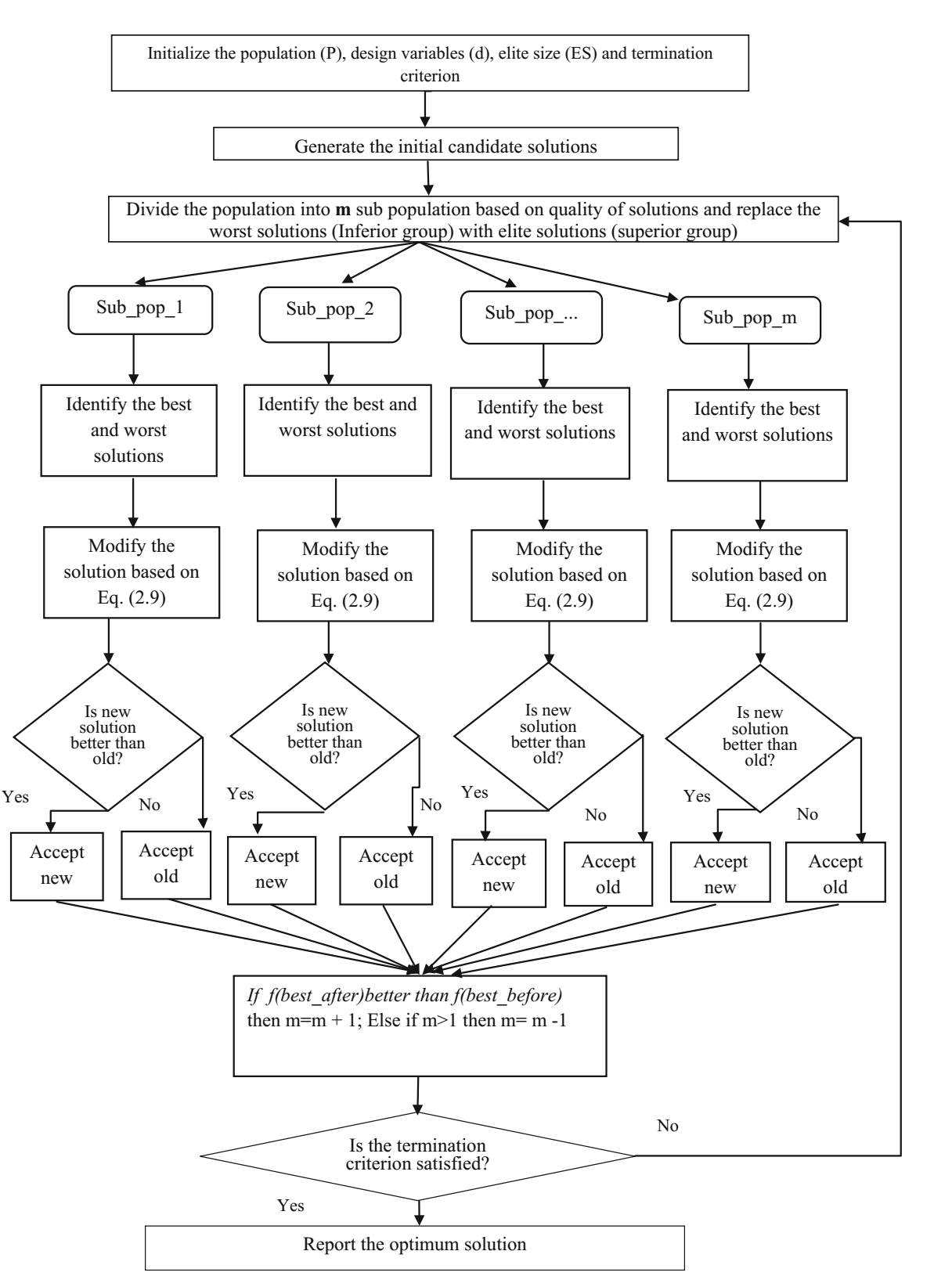## 多目标 Jaya（MO-Jaya）算法

MO-Jaya 算法是为解决多目标优化问题而开发的 (Rao et al. 2017)。MO-Jaya 算法是求解多目标优化问题的 Jaya 算法的后验版本。MO-Jaya 算法中解以类似于 Jaya 算法中等式 (9) 的方式更新。然而，为了有效地处理多个目标，MO-Jaya 算法结合了非支配排序方法和拥挤距离计算机制。非支配排序和拥挤距离机制的概念已在第 2 节中解释过。### 约束双目标优化问题的 MO-Jaya 算法演示

parameters）的双目标优化问题。Yang and Natarajan (2010) 用差分进化和非支配排序遗传算法-II解决了这个问题。该问题有两个目标：最小化刀具磨损 $T_w$ 和最大化金属去除率 $M_r$。目标函数、约束条件和切削参数范围如下。

• 温度约束：
• 表面粗糙度约束：
• 参数界限：

$v$ = 速度 (米/分钟)；$f$ = 进料 (毫米/转)；$d$ = 切割深度 (毫米)；$M_r$ = 金属去除率 (毫米/分钟)；$T_w$ = 刀具磨损 (毫米)；$T$ = 刀-工界面温度 (°C)；$R_a$ = 表面粗糙度 (微米)。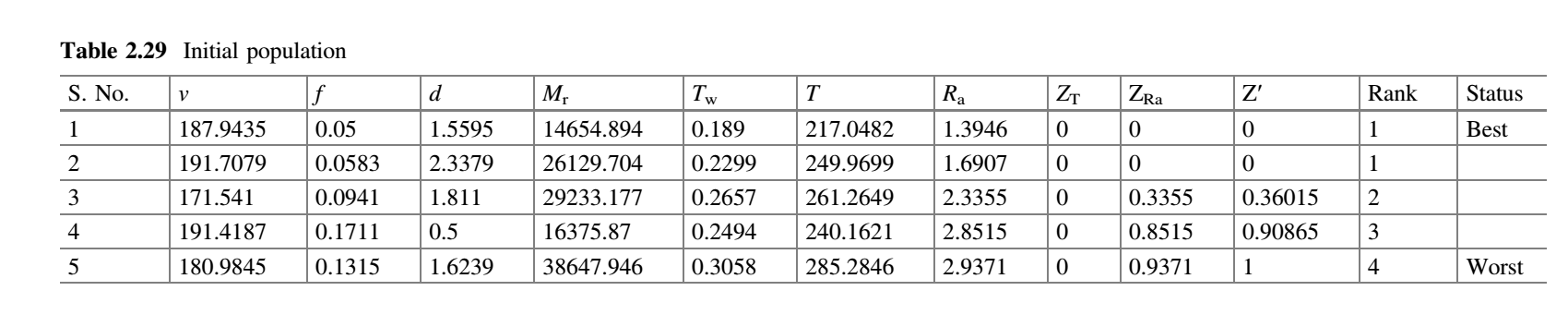Z’ = 整体约束冲突，可以写成，

$v$、$f$$d$ 的随机数 $r_1$$r_2$ 可以取 0.54 和 0.29；0.48 和 0.37；0.62 和 0.14。用等式 (9) 计算 $v$$f$$d$ 的新值。表 2.30 显示了 $v$$f$$d$ 的新值以及目标函数的相应值和约束值。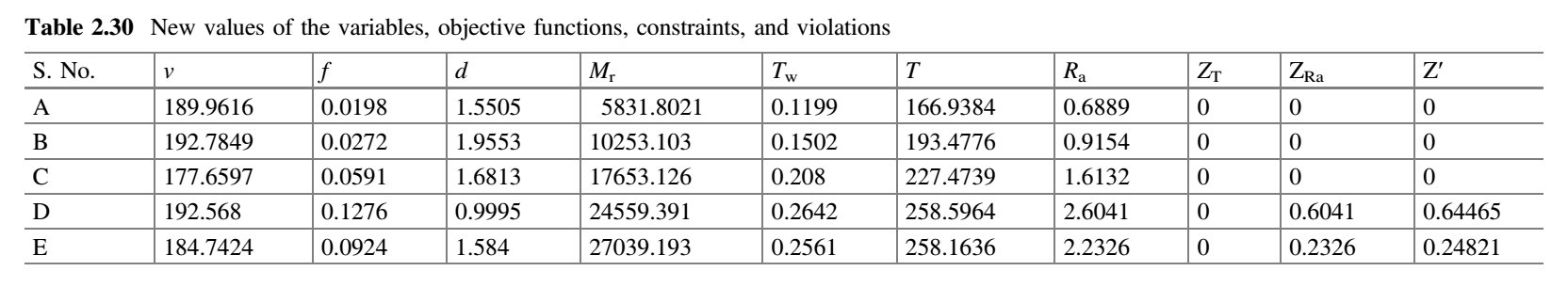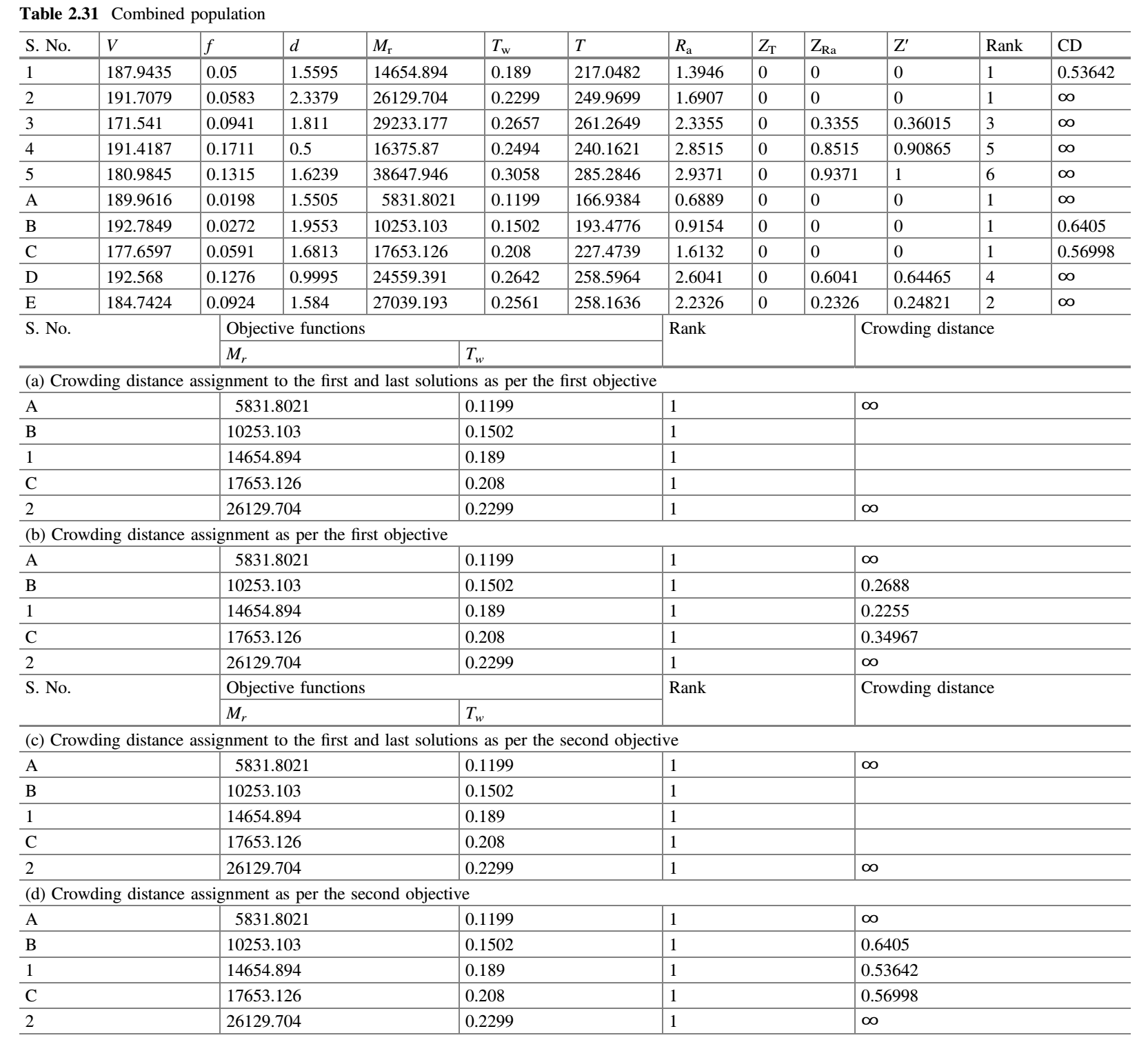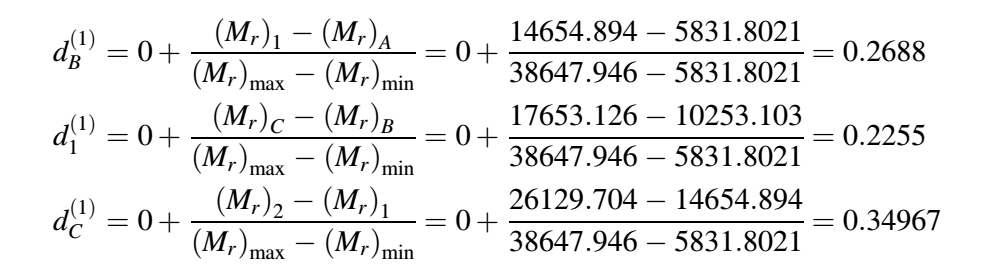1. 根据约束-支配和非支配排序概念对表 2.31 的种群进行排序。
2. 收集所有秩为 1 的解。
3. 从表 2.31 中确定整个种群的两个目标函数的最小值和最大值，这两个值是：$(M_r) _ {min} = 589.8021$$(M_r) _ {max} = 52967.946$$(T_w) _ {min} = 0.1199$$(T_w) _ {max} = 0.3321$
4. 只考虑第一个目标，并按升序对第一个目标函数的所有值进行排序。
1. 将第一个和最后一个解（即最佳和最差解）的拥挤距离分配为无穷，如表 2.31a 所示。
2. 拥挤距离 $d_B$$d_1$$d_C$ 计算如上图所示。表 2.31b 显示了根据第一个目标分配的拥挤距离。
5. 只考虑第二个目标，按升序对第二个目标函数值进行排序。
1. 将第一个和最后一个解（即最佳和最差解）的拥挤距离分配为无穷，如表 2.31c 所示。
2. 拥挤距离 $d_B$$d_1$$d_C$ 计算如下图所示。表 2.31d 显示了根据第二个目标分配的拥挤距离。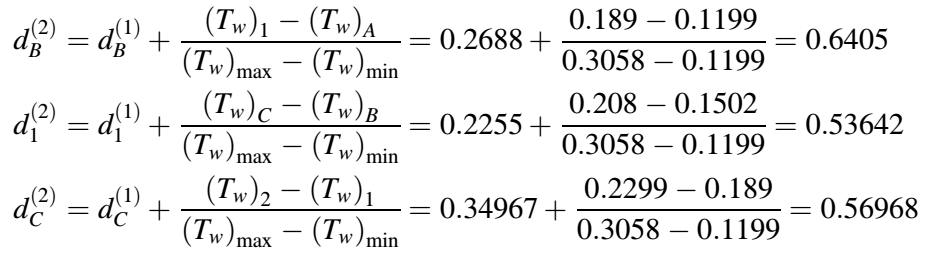## 多目标拟反向 Jaya（MOQO - Jaya）算法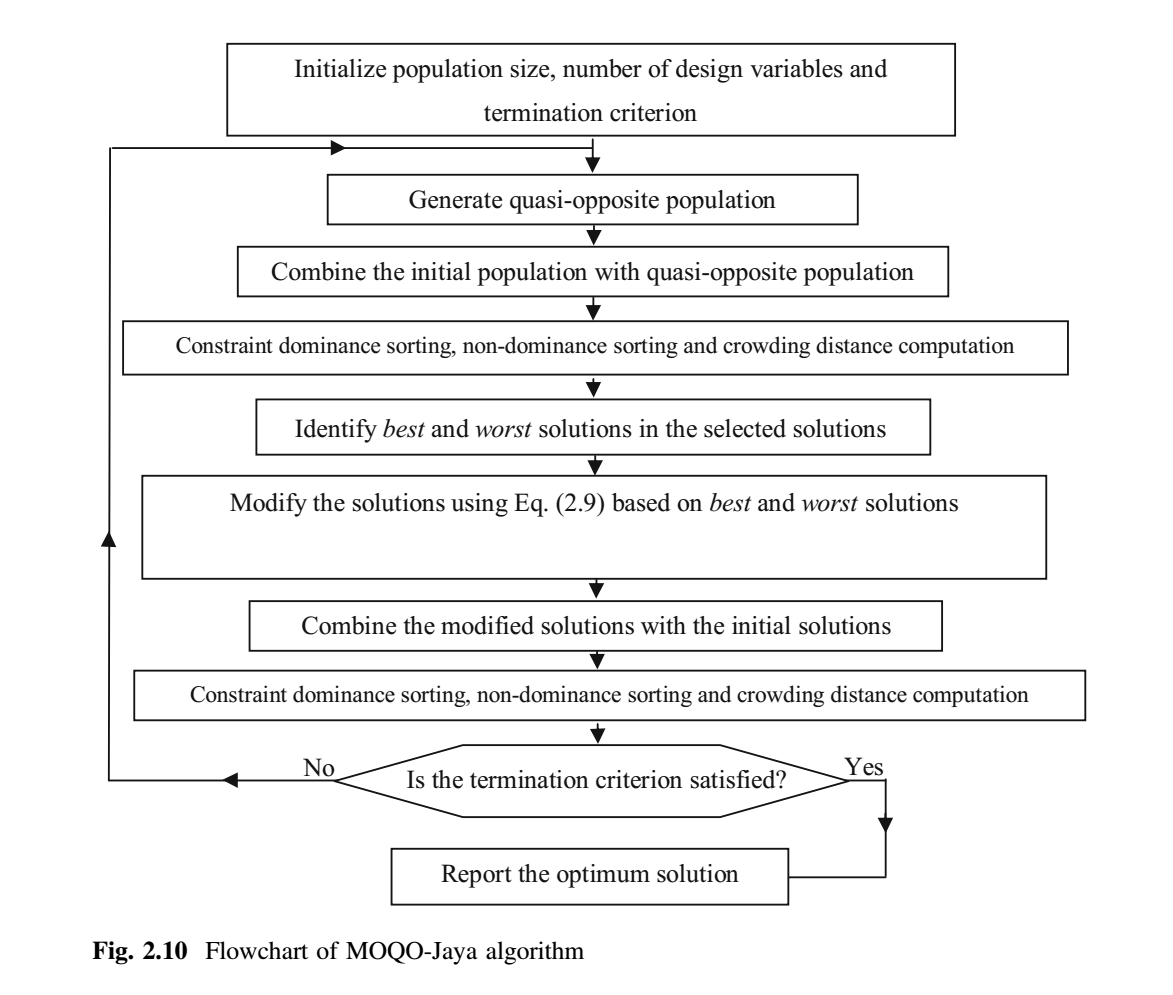## 性能评估

### 覆盖率（Coverage）

Cov (A, B) = 1 表示 B 中的所有点由 A 中的所有点支配或等于 A 中所有的点，$Cov(A, B) = 0$ 表示 B 中的所有解都不被集合 A 覆盖的情况。这里，必须同时考虑 $Cov(A, B)$$Cov(B, A)$，因为 $Cov(A, B)$ 不一定等于 $1-Cov(B, A)$。当 $Cov(A, B) = 1$$Cov(B, A) = 0$ 时，A 中的解完全支配 B 中的解（这即 A 的最佳可能性能）。$Cov(A, B)$ 表示 B 中解劣于或等于 A 中的解的百分比；$Cov(B, A)$ 表示 A 解劣于或等于 B 中的解的百分比。

### 超体积（Hypervolume）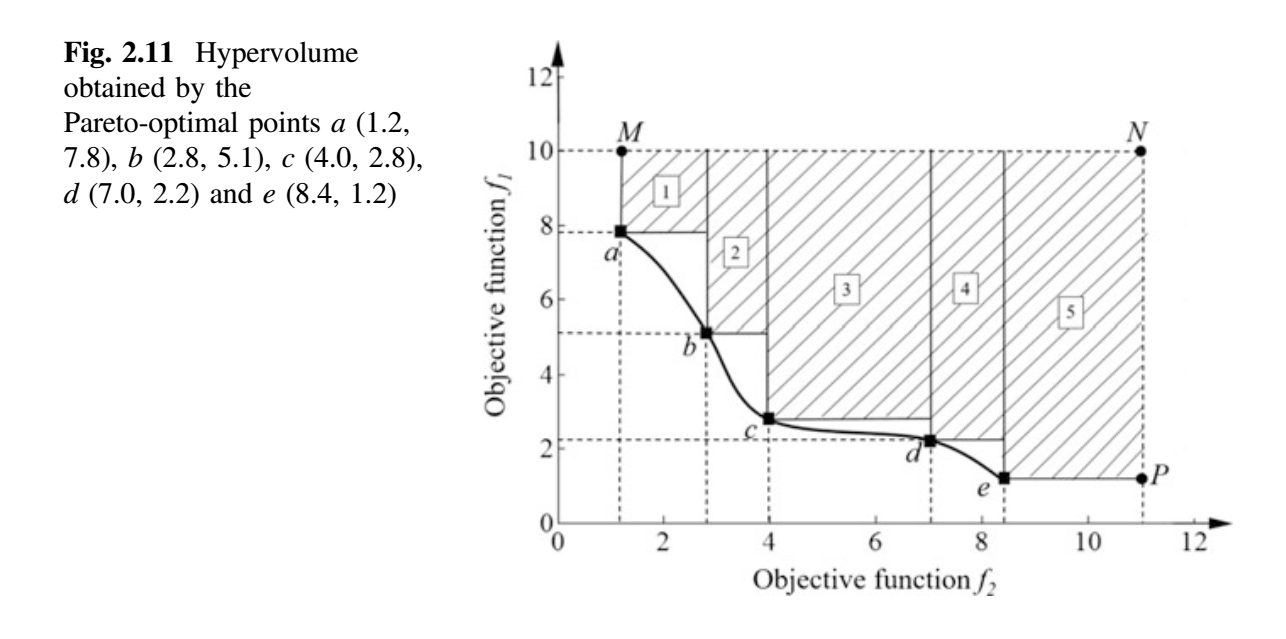Beume, N., Fonseca, C. M., Manuel, L.-I., Paquete, L., & Vahrenhold, J. (2009). On the complexity of computing the hypervolume indicator. IEEE Transactions on Evolutionary Computation, 13(5), 1075–1082.

Jiang, S., Zhang, J., Ong, Y.-S., Zhang, A. N., & Tan, P. S. (2015). A simple and fast hypervolume indicator-based multiobjective evolutionary algorithm. IEEE Transactions on Cybernetics, 45 (10), 2202–2213.

Rao, R. V. (2016a). Teaching learning based optimization algorithm and its engineering applications. Switzerland: Springer.

Rao, R. V. (2016b). Jaya: A simple and new optimization algorithm for solving constrained and unconstrained optimization problems. International Journal of Industrial Engineering Computations, 7, 19–34.

Rao, R. V., & More, K. (2017a). Design optimization and analysis of selected thermal devices using self-adaptive Jayaalgorithm. Energy Conversion and Management, 140, 24–35.

Rao, R. V., & More, K. (2017b). Optimal design and analysis of mechanical draft cooling tower using improved Jaya algorithm. International Journal of Refrigeration. https://doi.org/10.1016/ j.ijrefrig.2017.06.024.

Rao, R. V., & Rai, D. P. (2017a). Optimization of welding processes using quasi oppositional based Jaya algorithm. Journal of Experimental & Theoretical Artiﬁcial Intelligence, 29(5), 1099–1117.

Rao, R. V., & Rai, D. P. (2017b). Optimization of submerged arc welding process using quasi-oppositional based Jaya algorithm. Journal of Mechanical Science and Technology, 31(5), 1–10.

Rao, R. V., Rai, D. P., Balic, J. (2016). Multi-objective optimization of machining and micro-machining processes using non-dominated sorting teaching–Learning-based optimiza- tion algorithm. Journal of Intelligent Manufacturing, 2016. https://doi.org/10.1007/s10845- 016-1210-5.

Rao, R. V., Rai, D. P., & Balic, J. (2017). A multi-objective algorithm for optimization of modern machining processes. Engineering Applications of Artiﬁcial Intelligence, 61, 103–125.

Rao, R. V., & Saroj, A. (2017). A self-adaptive multi-population based Jaya algorithm for engineering optimization. Swarm and Evolutionary Computation. https://doi.org/10.1016/j. swevo.2017.04.008.

Rao, R. V., & Saroj, A. (2018). An elitism-based self-adaptive multi-population Jaya algorithm and its applications. Soft Computing. https://doi.org/10.1007/s00500-018-3095-z.

Rao, R. V., Savsani, V. J., & Vakharia, D. P. (2011). Teaching–learning-based optimization: A novel method for constrained mechanical design optimization problems. Computer-Aided Design, 43, 303–315. Simon, D. (2013). Evolutionary optimization algorithms. New York: Wiley.

Teo, T. (2006). Exploring dynamic self-adaptive populations in differential evolution. Soft Computing, 10, 673–686.

Yang, S. H., & Natarajan, U. (2010). Multiobjective optimization of cutting parameters in turning process using differential evolution and non-dominated sorting genetic algorithm-II approaches. International Journal of Advanced Manufacturing Technology, 49, 773–784.

Zhou, A., Qu, B.-Y., Li, H., Zhao, S.-Z., Suganthan, P. N., & Zhang, Q. (2011). Multiobjective evolutionary algorithms: a survey of the state of the art. Swarm and Evolutionary Computation, 1(1), 32–49.

## 附录：MATLAB 代码

### MOQO Jaya Code for ECM Process